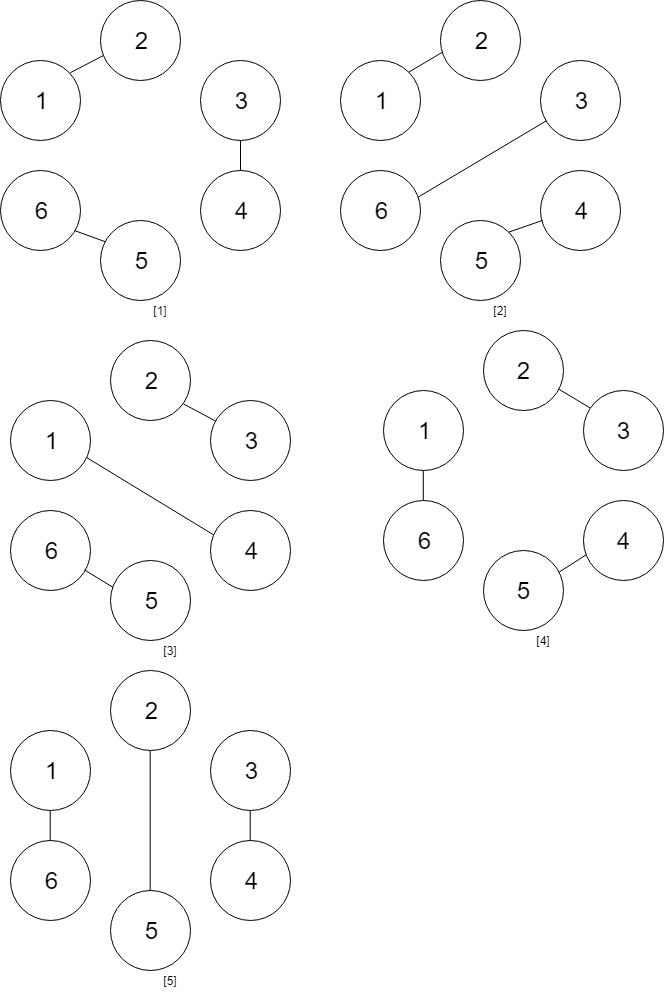##### Welcome to Subscribe On Youtube

Formatted question description: https://leetcode.ca/all/1259.html

# 1259. Handshakes That Don’t Cross

Hard

## Description

You are given an even number of people num_people that stand around a circle and each person shakes hands with someone else, so that there are num_people / 2 handshakes total.

Return the number of ways these handshakes could occur such that none of the handshakes cross.

Since this number could be very big, return the answer mod 10^9 + 7.

Example 1:

Input: num_people = 2

Output: 1

Example 2:Input: num_people = 4

Output: 2

Explanation: There are two ways to do it, the first way is [(1,2),(3,4)] and the second one is [(2,3),(4,1)].

Example 3:Input: num_people = 6

Output: 5

Example 4:

Input: num_people = 8

Output: 14

Constraints:

• 2 <= num_people <= 1000
• num_people % 2 == 0

## Solution

Use dynamic programming. Consider the number of pairs, which equals half the number of people. That is, let numPairs = num_people / 2. Create an array dp of length numPairs + 1, where dp[i] represents the number of ways of i pairs of people’s handshakes such that none of the handshakes cross. Initialize dp = 1 and dp = 1. If there are two people, which is equivalent to one pair, then obviously there is one handshake and one way. If there are no people, then there is no handshake, which is also one way.

For i from 2 to numPairs, suppose the people are labelled from 1 to 2 * numPairs, and consider the person with label 1, who can only shake hands with people with even labels to make sure none of the handshakes cross. Suppose the person with label 1 shakes hands with the person with label n, then there must be an even number of people in the label range 2 to n - 1 and there must be an even number of people in the label range n + 1 to 2 * numPairs, so n must be an even number. For each even number n, since the person with label 1 and the person with label n shake hands, the rest of the people are divided into two groups, which have n - 2 people and 2 * numPairs - n people respectively. Therefore, for each j from 0 to i - 1, update dp[i] = dp[i] + dp[j] * dp[i - j - 1]. Remember to do the mod operation on each element of dp. Finally, return dp[numPairs].

• class Solution {
public int numberOfWays(int num_people) {
int numPairs = num_people / 2;
if (numPairs == 0 || numPairs == 1)
return 1;
final int MODULO = 1000000007;
long[] dp = new long[numPairs + 1];
dp = 1;
dp = 1;
for (int i = 2; i <= numPairs; i++) {
for (int j = 0; j < i; j++)
dp[i] = (dp[i] + dp[j] * dp[i - j - 1]) % MODULO;
}
return (int) (dp[numPairs] % MODULO);
}
}